GATE  >  Chemistry- CY 2014 GATE Paper (Practice Test)

# Chemistry- CY 2014 GATE Paper (Practice Test)

Test Description

## 65 Questions MCQ Test GATE Past Year Papers for Practice (All Branches) | Chemistry- CY 2014 GATE Paper (Practice Test)

Chemistry- CY 2014 GATE Paper (Practice Test) for GATE 2022 is part of GATE Past Year Papers for Practice (All Branches) preparation. The Chemistry- CY 2014 GATE Paper (Practice Test) questions and answers have been prepared according to the GATE exam syllabus.The Chemistry- CY 2014 GATE Paper (Practice Test) MCQs are made for GATE 2022 Exam. Find important definitions, questions, notes, meanings, examples, exercises, MCQs and online tests for Chemistry- CY 2014 GATE Paper (Practice Test) below.
Solutions of Chemistry- CY 2014 GATE Paper (Practice Test) questions in English are available as part of our GATE Past Year Papers for Practice (All Branches) for GATE & Chemistry- CY 2014 GATE Paper (Practice Test) solutions in Hindi for GATE Past Year Papers for Practice (All Branches) course. Download more important topics, notes, lectures and mock test series for GATE Exam by signing up for free. Attempt Chemistry- CY 2014 GATE Paper (Practice Test) | 65 questions in 180 minutes | Mock test for GATE preparation | Free important questions MCQ to study GATE Past Year Papers for Practice (All Branches) for GATE Exam | Download free PDF with solutions
 1 Crore+ students have signed up on EduRev. Have you?
Chemistry- CY 2014 GATE Paper (Practice Test) - Question 1

### Q. 1 – Q. 5 carry one mark each. Q A student is required to demonstrate a high level of comprehension of the subject, especially in the social sciences The word closest in meaning to comprehension is

Chemistry- CY 2014 GATE Paper (Practice Test) - Question 2

### Choose the most appropriate word from the options given below to complete the following sentence. One of his biggest ______ was his ability to forgive.

Chemistry- CY 2014 GATE Paper (Practice Test) - Question 3

### Rajan was not happy that Sajan decided to do the project on his own. On observing his unhappiness, Sajan explained to Rajan that he preferred to work independently. Which one of the statements below is logically valid and can be inferred from the above sentences?

Chemistry- CY 2014 GATE Paper (Practice Test) - Question 4

If y = 5x2 + 3, then the tangent at x = 0, y = 3

*Answer can only contain numeric values
Chemistry- CY 2014 GATE Paper (Practice Test) - Question 5

A foundry has a fixed daily cost of Rs 50,000 whenever it operates and a variable cost of Rs 800Q,
where Q is the daily production in tonnes. What is the cost of production in Rs per tonne for a daily
production of 100 tonnes?

Important : you should answer only the numeric value

Chemistry- CY 2014 GATE Paper (Practice Test) - Question 6

Q. 6 – Q. 10 carry two marks each

Q.

Find the odd one in the following group: ALRVX, EPVZB, ITZDF, OYEIK

Chemistry- CY 2014 GATE Paper (Practice Test) - Question 7

Anuj, Bhola, Chandan, Dilip, Eswar and Faisal live on different floors in a six-storeyed building
(the ground floor is numbered 1, the floor above it 2, and so on). Anuj lives on an even-numbered
floor. Bhola does not live on an odd numbered floor. Chandan does not live on any of the floors
below Faisal’s floor. Dilip does not live on floor number 2. Eswar does not live on a floor
immediately above or immediately below Bhola. Faisal lives three floors above Dilip. Which of the
following floor-person combinations is correct?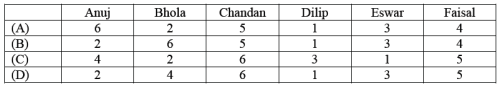*Answer can only contain numeric values
Chemistry- CY 2014 GATE Paper (Practice Test) - Question 8

The smallest angle of a triangle is equal to two thirds of the smallest angle of a quadrilateral. The
ratio between the angles of the quadrilateral is 3:4:5:6. The largest angle of the triangle is twice its
smallest angle. What is the sum, in degrees, of the second largest angle of the triangle and the

Important : you should answer only the numeric value

Chemistry- CY 2014 GATE Paper (Practice Test) - Question 9

One percent of the people of country X are taller than 6 ft. Two percent of the people of country Y
are taller than 6 ft. There are thrice as many people in country X as in country Y. Taking both
countries together, what is the percentage of people taller than 6 ft?

Chemistry- CY 2014 GATE Paper (Practice Test) - Question 10

The monthly rainfall chart based on 50 years of rainfall in Agra is shown in the following figure.
Which of the following are true? (k percentile is the value such that k percent of the data fall below
that value)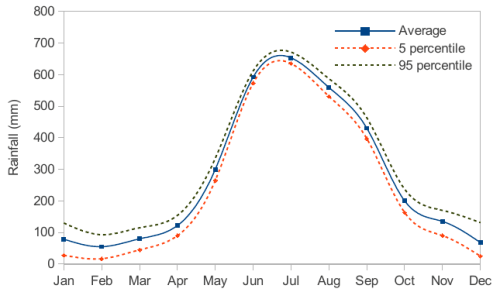(i) On average, it rains more in July than in December
(ii) Every year, the amount of rainfall in August is more than that in January
(iii) July rainfall can be estimated with better confidence than February rainfall
(iv) In August, there is at least 500 mm of rainfall

Chemistry- CY 2014 GATE Paper (Practice Test) - Question 11

Q. 11 – Q. 35 carry one mark each.

Q.

The maximum non-PV work that a system can perform at constant P is

Chemistry- CY 2014 GATE Paper (Practice Test) - Question 12

Consider the reaction:
A + B⇔ C
The unit of the thermodynamic equilibrium constant for the reaction is

*Answer can only contain numeric values
Chemistry- CY 2014 GATE Paper (Practice Test) - Question 13

The number of IR active vibrational normal modes of CO2 is _____________

Important : you should answer only the numeric value

*Answer can only contain numeric values
Chemistry- CY 2014 GATE Paper (Practice Test) - Question 14

The number of C2 axes in CCl4 is ______________

Important : you should answer only the numeric value

Chemistry- CY 2014 GATE Paper (Practice Test) - Question 15

The value of the magnetic quantum number of a px orbital is

Chemistry- CY 2014 GATE Paper (Practice Test) - Question 16

The molecular partition function for a system in which the energy levels are equispaced by ε , is

Chemistry- CY 2014 GATE Paper (Practice Test) - Question 17

A monoatomic gas, X, adsorbed on a surface, follows Langmuir adsorption isotherm. A plot of the
fraction of surface coverage, θ, against the concentration of the gas [X], for VERY LOW
concentration of the gas, is described by the equation

*Answer can only contain numeric values
Chemistry- CY 2014 GATE Paper (Practice Test) - Question 18

At a given temperature and pressure, the ratio of the average speed of hydrogen gas to that of
helium gas is approximately ______________

Chemistry- CY 2014 GATE Paper (Practice Test) - Question 19

An example of nido-borane from the following is

Chemistry- CY 2014 GATE Paper (Practice Test) - Question 20

The geometries of Ni(CO)4 and [NiCl4]2−, respectively, are

*Answer can only contain numeric values
Chemistry- CY 2014 GATE Paper (Practice Test) - Question 21

The number of S−S bonds in H2S5O6 is ______________

Important : you should answer only the numeric value

Chemistry- CY 2014 GATE Paper (Practice Test) - Question 22

In atomic absorption spectroscopy, the atomization process utilizes

*Answer can only contain numeric values
Chemistry- CY 2014 GATE Paper (Practice Test) - Question 23

At room temperature, the number of singlet resonances observed in the 1H NMR spectrum of
Me3CC(O)NMe2 (N,N-dimethyl pivalamide) is ______________

Important : you should answer only the numeric value

Chemistry- CY 2014 GATE Paper (Practice Test) - Question 24

Amongst the following, the metal that does NOT form homoleptic polynuclear metal carbonyl is

Chemistry- CY 2014 GATE Paper (Practice Test) - Question 25

The reaction of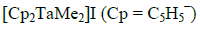with NaOMe yields

Chemistry- CY 2014 GATE Paper (Practice Test) - Question 26

The complexes [Co(H2O)4Cl2]NO2 and [Co(H2O)4Cl(NO2)]Cl are

Chemistry- CY 2014 GATE Paper (Practice Test) - Question 27

The major product of the following reaction is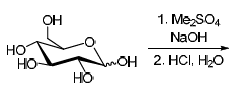Chemistry- CY 2014 GATE Paper (Practice Test) - Question 28

Amongst the following, the structure of guanosine is

Chemistry- CY 2014 GATE Paper (Practice Test) - Question 29

The correct order of IR stretching frequency of the C=C in the following olefins is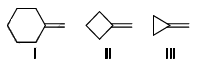Chemistry- CY 2014 GATE Paper (Practice Test) - Question 30

The correct order of the rate of solvolysis for the following chlorides in acetic acid is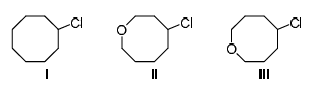Chemistry- CY 2014 GATE Paper (Practice Test) - Question 31

Formation of the product in the following photochemical reaction involves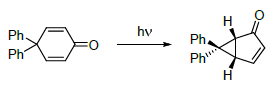Chemistry- CY 2014 GATE Paper (Practice Test) - Question 32

The correct order of stability for the following conformations of cyclohexane is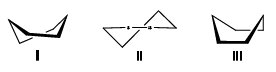Chemistry- CY 2014 GATE Paper (Practice Test) - Question 33

The major product formed in the following reaction is*Answer can only contain numeric values
Chemistry- CY 2014 GATE Paper (Practice Test) - Question 34

The overall yield (in %) for the following reaction sequence is ______________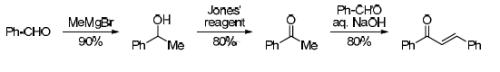Chemistry- CY 2014 GATE Paper (Practice Test) - Question 35

The most suitable reagent combination to effect the following conversion is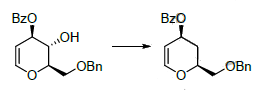Chemistry- CY 2014 GATE Paper (Practice Test) - Question 36

Q. 26 – Q. 55 carry two marks each.

Q.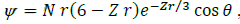is a proposed hydrogenic wavefunction, where Z = Atomic
number, r = radial distance from the nucleus, θ = azimuthal angle, N is a constant. The

*Answer can only contain numeric values
Chemistry- CY 2014 GATE Paper (Practice Test) - Question 37

The van der Waals constants a and b of CO2 are 3.64 L2 bar mol−2 and 0.04 L mol−1, respectively.
The value of R is 0.083 bar dm3 mol−1 K−1. If one mole of CO2 is confined to a volume of 0.15 L at
300 K, then the pressure (in bar) exerted by the gas, is ______________

Chemistry- CY 2014 GATE Paper (Practice Test) - Question 38

A plot pf osmotic pressure against concentration (g L-1) of a polymer is constructed. The slop of the plot

*Answer can only contain numeric values
Chemistry- CY 2014 GATE Paper (Practice Test) - Question 39

A platinum electrode is im mersed in a solution containing 0.1 M Fe2+ and 0.1 M Fe3+. Its potential is found to be 0.77 V against SHE. Und er standard conditions and considering activity coefficients to be equal to unity, the potential of the electrode, when the con centration of Fe3+ is incre ased to 1 M , is ______________

*Answer can only contain numeric values
Chemistry- CY 2014 GATE Paper (Practice Test) - Question 40

Molybdenum crystallizes in a bcc structure with unit cell dimensions of  0.314  nm. Considering the

atomic mass of molybdenum to be 96, its density (in kg m3)  is

*Answer can only contain numeric values
Chemistry- CY 2014 GATE Paper (Practice Test) - Question 41

The ratio of molecules distributed between two states is 9.22 ×106 energy (in kJ m ol1) of the two states is __________ ____

*Answer can only contain numeric values
Chemistry- CY 2014 GATE Paper (Practice Test) - Question 42

A Carnot engine operates at 55% efficiency. If the temperature o f reject steam is 105 oC, then the

absolute temperature of input steam is ___________ ___

Chemistry- CY 2014 GATE Paper (Practice Test) - Question 43

Of the following plots, the correct representation of chemical potential (µ against absolute temperature (T) for a pure substance is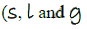denote solid, liquid and gas phases, respectively)

*Answer can only contain numeric values
Chemistry- CY 2014 GATE Paper (Practice Test) - Question 44

The enthalpy of fusion of ice at 273 K is 6.01 kJ mol−1 and the enthalpy of vaporization of water at 27 3 K is 44.83 kJ mol −1. The enthalpy of sublimation (in kJ mol1) of ice at 273 K, is __ _________

*Answer can only contain numeric values
Chemistry- CY 2014 GATE Paper (Practice Test) - Question 45

Su ppose ψ1 and ψ2, are two hybrid orbi tals: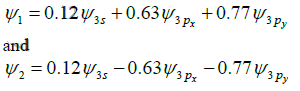The angle (in degrees) between them is __________

Chemistry- CY 2014 GATE Paper (Practice Test) - Question 46

BCl3 and NH4C l were heate d at 140 oC to give compound X, which when tre ated with NaBH4 gave ano ther compo und Y. Com pounds X a nd Y are

*Answer can only contain numeric values
Chemistry- CY 2014 GATE Paper (Practice Test) - Question 47

The number of microstates in term 1G is ______________

Important : you should answer only the numeric value

Chemistry- CY 2014 GATE Paper (Practice Test) - Question 48

The set of protons (underlined) in CH3CH2CH2OCH3 that would exhibit different splitting patterns
in high (500 MHz) and low (60 MHz) field 1H NMR, is

Chemistry- CY 2014 GATE Paper (Practice Test) - Question 49

Amongst the following, the complex ion that would show strong Jahn-Teller distortion is

Chemistry- CY 2014 GATE Paper (Practice Test) - Question 50

Amongst the following, the metal carbonyl species having the highest νCO stretching frequency is

Chemistry- CY 2014 GATE Paper (Practice Test) - Question 51

The correct order of thermal stability for the given compounds is

Chemistry- CY 2014 GATE Paper (Practice Test) - Question 52

Amongst the following, the complex ion that is expected to show the highest magnetic moment at
room temperature is

Chemistry- CY 2014 GATE Paper (Practice Test) - Question 53

MnCr2O4 is

Chemistry- CY 2014 GATE Paper (Practice Test) - Question 54

Mg2+ is preferred in photosynthesis by chlorophyll because

Chemistry- CY 2014 GATE Paper (Practice Test) - Question 55

In Monsanto acetic acid process shown below, the role of HI is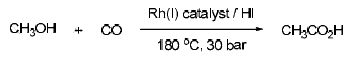Chemistry- CY 2014 GATE Paper (Practice Test) - Question 56

Formation of the ketone II from the diazoketone I involves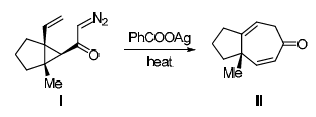Chemistry- CY 2014 GATE Paper (Practice Test) - Question 57

The major products X and Y formed in the following reaction sequence areChemistry- CY 2014 GATE Paper (Practice Test) - Question 58

The major products X and Y formed in the following reactions are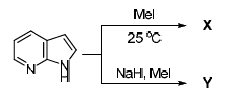Chemistry- CY 2014 GATE Paper (Practice Test) - Question 59

The major products X and Y formed in the following reaction sequence are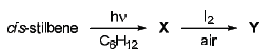Chemistry- CY 2014 GATE Paper (Practice Test) - Question 60

The product of the following reaction gave 6 line 13C NMR spectrum with peaks at δ 175, 52, 50, 46, 37, 33 ppm. The structure of the product is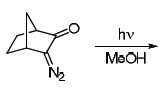Chemistry- CY 2014 GATE Paper (Practice Test) - Question 61

The major product formed in the following reaction is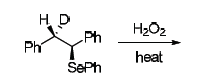Chemistry- CY 2014 GATE Paper (Practice Test) - Question 62

The major products X and Y formed in the following reaction sequence are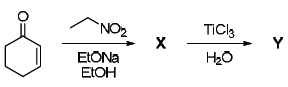Chemistry- CY 2014 GATE Paper (Practice Test) - Question 63

The major products X and Y formed in the following reaction sequence are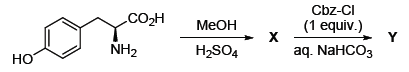*Answer can only contain numeric values
Chemistry- CY 2014 GATE Paper (Practice Test) - Question 64

Given the fact that 1,3-butadiene has a UV absorption of 17 nm, the absorption wavelength (in nm) for the conjugated system shown below is ______________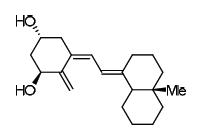(Use these absorption values for auxochromic groups:

alkyl: +5; exo-cyclic double bond: +5; every additional conjugated C=C: +30)

Important : you should answer only the numeric value

*Answer can only contain numeric values
Chemistry- CY 2014 GATE Paper (Practice Test) - Question 65

The m/z value of the detectable fragment formed by McLafferty like rearrangement of the following compound in mass spectrometer is ______________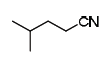Important : you should answer only the numeric value

## GATE Past Year Papers for Practice (All Branches)

380 docs|127 tests
 Use Code STAYHOME200 and get INR 200 additional OFF Use Coupon Code
Information about Chemistry- CY 2014 GATE Paper (Practice Test) Page
In this test you can find the Exam questions for Chemistry- CY 2014 GATE Paper (Practice Test) solved & explained in the simplest way possible. Besides giving Questions and answers for Chemistry- CY 2014 GATE Paper (Practice Test), EduRev gives you an ample number of Online tests for practice

## GATE Past Year Papers for Practice (All Branches)

380 docs|127 tests